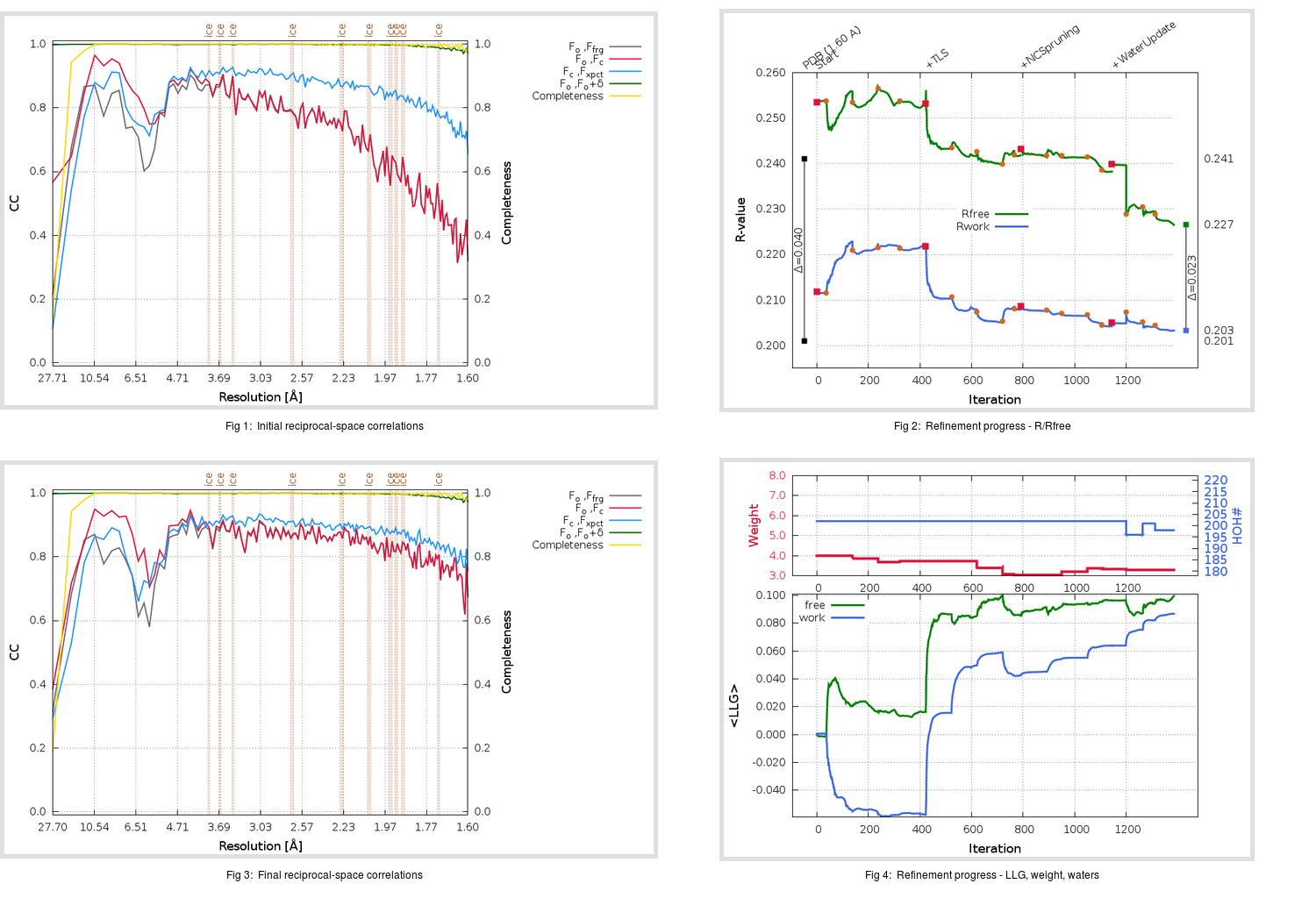Content:

```    Diffraction limits & principal axes of ellipsoid fitted to diffraction cut-off surface:
1.579         0.8054   0.0000   0.5927       0.992 a* + 0.124 c*
1.543         0.0000   1.0000   0.0000       b*
1.565        -0.5927   0.0000   0.8054      -0.709 a* + 0.706 c*
```

## Deposited

` `
 Date deposited Date data collection Resolution R, Rfree 20041129 20040202 1.60 0.1990 0.2410

Molprobity (CCP4 7.0 version) summary:

```Ramachandran outliers =   0.00 %
favored =  98.29 %
Rotamer outliers      =   7.73 %
C-beta deviations     =     0
Clashscore            =   2.59
RMS(bonds)            =   0.0168
RMS(angles)           =   1.82
MolProbity score      =   1.72
Resolution            =   1.60
R-work                =   0.1990
R-free                =   0.2410
```

```Number of waters      =   202

<B> (all atoms) =   34.66 ( sd =    9.07 ) for       2110 non-hydrogen atoms
<B>   (protein) =   33.51 ( sd =    8.28 ) for       1890 non-hydrogen atoms
<B>     (water) =   45.36 ( sd =    9.90 ) for        202 non-hydrogen atoms
<B>    (others) =   38.16 ( sd =    5.39 ) for         18 non-hydrogen atoms

B min/max       (all non-hydrogen atoms) =   19.15 /   68.21
B min/max   (protein non-hydrogen atoms) =   19.15 /   61.39
B min/max     (water non-hydrogen atoms) =   22.22 /   68.21
B min/max     (other non-hydrogen atoms) =   27.43 /   50.88
```

## BUSTER (re-)refinement

` `

Molprobity (CCP4 7.0 version) summary:

```Ramachandran outliers =   0.43 %
favored =  99.15 %
Rotamer outliers      =   3.64 %
C-beta deviations     =     0
Clashscore            =   3.11
RMS(bonds)            =   0.0110
RMS(angles)           =   1.32
MolProbity score      =   1.53
Resolution            =   1.60
R-work                =   0.2033
R-free                =   0.2265
```

```Number of waters      =   198

<B> (all atoms) =   37.55 ( sd =   10.99 ) for       2106 non-hydrogen atoms
<B>   (protein) =   36.21 ( sd =   10.17 ) for       1890 non-hydrogen atoms
<B>     (water) =   50.11 ( sd =   11.35 ) for        198 non-hydrogen atoms
<B>    (others) =   42.97 ( sd =    6.04 ) for         18 non-hydrogen atoms

B min/max       (all non-hydrogen atoms) =   20.81 /   75.95
B min/max   (protein non-hydrogen atoms) =   20.81 /   71.40
B min/max     (water non-hydrogen atoms) =   23.65 /   75.95
B min/max     (other non-hydrogen atoms) =   32.86 /   53.17
```

Refinement progression:Results:

` `
 File Remark 2BEQ_aB_refine.01_04_refine.pdb.gz exact refinement commands are in header 2BEQ_aB_refine.01_04_refine.mtz.gz including original deposited data and several re-refinement map coefficients 2BEQ_aB_refine.01_04_BUSTER_model.cif.gz including any non-standard compound restraints 2BEQ_aB_refine.01_04_BUSTER_refln.cif.gz